Courses

# Chemistry Test 1 - Mole Concept, Atomic Structure, Bonding, Periodic Properties, General Organic Compounds

## 30 Questions MCQ Test JEE Main Mock Test Series 2020 & Previous Year Papers | Chemistry Test 1 - Mole Concept, Atomic Structure, Bonding, Periodic Properties, General Organic Compounds

Description
This mock test of Chemistry Test 1 - Mole Concept, Atomic Structure, Bonding, Periodic Properties, General Organic Compounds for JEE helps you for every JEE entrance exam. This contains 30 Multiple Choice Questions for JEE Chemistry Test 1 - Mole Concept, Atomic Structure, Bonding, Periodic Properties, General Organic Compounds (mcq) to study with solutions a complete question bank. The solved questions answers in this Chemistry Test 1 - Mole Concept, Atomic Structure, Bonding, Periodic Properties, General Organic Compounds quiz give you a good mix of easy questions and tough questions. JEE students definitely take this Chemistry Test 1 - Mole Concept, Atomic Structure, Bonding, Periodic Properties, General Organic Compounds exercise for a better result in the exam. You can find other Chemistry Test 1 - Mole Concept, Atomic Structure, Bonding, Periodic Properties, General Organic Compounds extra questions, long questions & short questions for JEE on EduRev as well by searching above.
QUESTION: 1

### 20 g of an ideal gas contains only atoms of S and O occupies 5.6 L at NPT. What is the mol. wt. of gas ?

Solution:

Given,
Mass of gas = 20 g
Pressure of gas = 1 atm
Volume of gas = 5.6 L
Temperature of gas = 273 K
Using ideal gas law,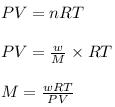where,
P = pressure of the gas
V = volume of the gas
T = temperature of the gas
n = number of moles of the gas
R = gas constant = 0.0821
Latm/moleK
M = molar mass of gas
w = mass of gas
Now put all the given values in the formula, we get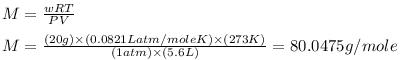Therefore, the molar mass of the gas is, 80.0475 g/mole

QUESTION: 2

### 5.6 litres of a gas at N.T.P. are found to have a mass of 11 g. The molecular mass of the gas is

Solution:
1 mole or molecular mass of the gas has volume 22.4 L
Given that:
11g of gas has volume 5.6 L

So molecular mass = (11/5.6)×22.4 = 44 u
QUESTION: 3

### A crystalline salt Na2SO4.xH2O on heating losses 55.9 per cent of its mass. The formula of crystalline salt is---

Solution: Na2SO4.xH2O has weight =46+32+64+18x=142+18xg
on heating it will become anhydrous so, I.e. water will be evaporated.
55.9% of(142+18x)=18x=>79.378+10.062x=18x
=>7.938x=79.378=>x~10g
so Na2SO4.10H2O and this amount of water is called
water of crystallisation.
QUESTION: 4

Vapour density of a metal chloride is 66. Its oxide contains 53% metal. The atomic weight of the metal is

Solution:
Equivalent of metal = Equivalent of chloride

53/n = 47/8 , E = 9.02 , Atomic mass of metal = n X 9.02

Molecular mass of chloride = 2 X 66 = n X 9.02 + n X 35.5

on solving n = 3

Atomic mass of metal = 9.02 X 3 = 27.06
QUESTION: 5

When two aqueous solutions react together to give off solid product, this reaction is known as

Solution:
Chemical reactions:precipitation. The precipitate forms because the solid (AgCl) is insoluble in water. That is true for all precipitates - the solids are insoluble in aqueous solutions. Precipitation reaction occur all around us.
QUESTION: 6

At S.T.P. the density of CCl4 vapour in g/L will be nearest to

Solution: Stp of mole of a gas = 22.4L 1mole --> 154g of CCl4 density=mass/volume =154/22.4 = 6.875
QUESTION: 7

Which of the following transitions of electrons in the hydrogen, atom will emit maximum energy ?

Solution:
QUESTION: 8

The uncertainty found from the uncertainty principle  (Δx.Δp = h/4 π) is

Solution:
Heisenberg uncertainty principle states that exact momentum and exact position of matter wave can't be determined simultaneously.
∆P.∆X>equal to h/4π
QUESTION: 9

The number of atoms of hydrogen in 2 moles of NH3

Solution:
QUESTION: 10

The spectrum of He is expected to be similar to that of

Solution:

The spectrum of an atom depends on the number of electrons present in it. Here, helium has two electrons, so the spectrum of Li+ (Z = 3) is similar to that of helium because both He and Li+ have two electrons.

QUESTION: 11

The electrons present in K-shell of the atom will differ in

Solution:
The electrons present in K-shell of the atom will differ in spin quantum number as the electrons will have opposite spins.
QUESTION: 12
Consider the following statements. The d-orbitals have
I. four lobes and two nodes
II. four lobes and one node
Which of the above statements are correct ?
Solution:
QUESTION: 13

Out of the two compounds shown below, the vapour pressing of B at a particular temperature is expected to be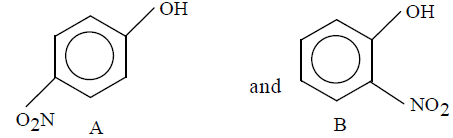Solution:
QUESTION: 14

In which of the following molecules, the central atom does not use sP3-hybrid orbitals in its bonding ?

Solution:
QUESTION: 15

The correct increasing bond angle among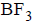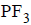and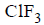Solution:
QUESTION: 16
Calculated bond order in the superoxide (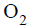) ion is
Solution: O2^- (superoxide) = 13 valence e⁻. = σs(2 e⁻) σs*(2e⁻) σp(2e⁻) πp(4e⁻) πp*(3e⁻) σp*(0) . bond order = ½[ σs(2e⁻) σp(2e⁻) πp(4 e⁻) - σs*(2e⁻) πp*(3e⁻) ]. = ½[8-5] = 1.5
QUESTION: 17

The ionic species having largest size is

Solution:
QUESTION: 18

From which of the following species it is easiest to remove one electron ?

Solution:
QUESTION: 19
With respect to chlorine, hydrogen will be
Solution:
With respect to Chlorine, hydrogen will be electropositive.

Reason:

Chlorine being a strong electronegative element tends to force hydrogen to lose electron and hydrogen acquire electropositive character so that chlorine can complete its octet.

QUESTION: 20

Which element has the greatest tendency to lose electrons ?

Solution: Fe has high electronegativity. it is a metal and it has proper to lose electron
QUESTION: 21
Which of the following atomic numbers represents an alkali metals ?
Solution: Because alkali metals have their valency 1 its general electronic configuration is ns^1
QUESTION: 22

Which of the following has highest solubility in water?

Solution:
QUESTION: 23

Which of the following decomposes at highest temperature ?

Solution:
QUESTION: 24

Nitrolim which is used as a fertilizer has the composition

Solution: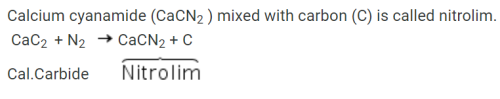It is also known as Lime-Nitrogen.

QUESTION: 25

The IPUAC name of the compound,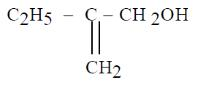is

Solution:
QUESTION: 26

IPUAC name of the compound,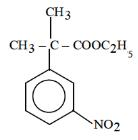is

Solution:
QUESTION: 27

The total number of structural isomers (acyclic and cyclic) possible for the molecular formula, C3H6 are

Solution:
QUESTION: 28

The stability of the compounds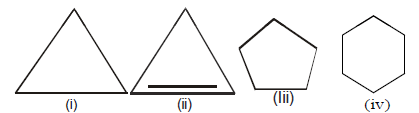Solution:
QUESTION: 29

The most stable free radical among the following is

Solution:
QUESTION: 30

Which of the following is correct regarding the-I-effect of the substituents ?

Solution: